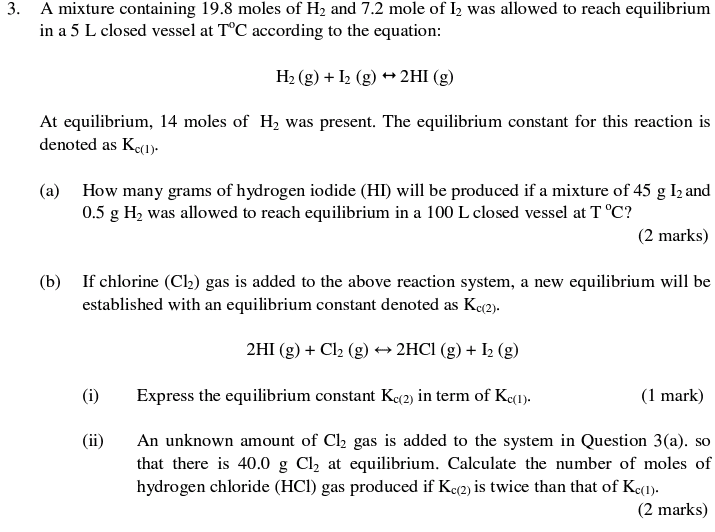# A complex equilibrium question

Member warned about not using the homework template
Kc1 = (5.8*2/5)^2 / (14/5)(1.4/5) = 6.865

6.865 = [HI]^2 / (45/253.8/100)(0.5/2/100)
[HI] = 5.52x10^-3 M
mass no of HI = [HI] x (126.9+1) x 100 = 70.6g
is it correct?
And how to do 3bii
I GOT 3bi Kc2 = [HCl(g)]^2/ / Kc(1) [H2(g)] [Cl2(g)]Bystander
Homework Helper
Gold Member
70.6g is it correct?
Think about it --- you've got 45 g I2 to start --- what's the largest mass of HI you can make?

6.865 = x^2 / ((45/253.8/100)-0.5x)((0.5/2/100)-0.5x)
This equation is OK?because the mole ratio of H2 to Hi is 1:2
So [HI]=x=5.33*10-4M
HI =6.813g?

Last edited:
Bystander
Homework Helper
Gold Member
The 6.865 is okay for an equilibrium constant. The notation you're using for the equation is unconventional. What're the "100s" doing in there?

The 6.865 is okay for an equilibrium constant. The notation you're using for the equation is unconventional. What're the "100s" doing in there?
100L
The volume

Bystander
Homework Helper
Gold Member
And the HI is not also occupying that volume?

Yes, but molarity = mole/volume
Does not volume is necessary?

Bystander
Homework Helper
Gold Member
You're using it in the denominator for hydrogen and iodine concentrations. Don't you think you should be using it in the numerator as well? After all, you did it that way when finding the equilibrium constant.

((45/253.8)/100)
？？this way？？

Bystander
Homework Helper
Gold Member
((45/253.8)/100)
？？this way？？
No, the way you did it for the very first step when you solved for the equilibrium constant.
Kc1 = (5.8*2/5)^2 / (14/5)(1.4/5) = 6.865

You're using it in the denominator for hydrogen and iodine concentrations
What it mean.....

Bystander
Homework Helper
Gold Member
Do you see where you inserted the number "5" for five liters in the very first calculation you did? Do you see it in both numerator, HI, and denominator, H and I?

Do you see where you inserted the number "5" for five liters in the very first calculation you did? Do you see it in both numerator, HI, and denominator, H and I?
For example
14 is the mole of h2,so 14/5=2.8 which is concentration of h2, right?
OK then there is 0.5g of h2,
0.5/2=0.25mol
0.25/100=1.25*10^-3M
What wrong with me?

Bystander
Homework Helper
Gold Member
6.865 =( x^2/1002) / ((45/253.8/100)-0.5x)((0.5/2/100)-0.5x)
So, you will have x moles of HI in 100 liters. Clear?

So, you will have x moles of HI in 100 liters. Clear?
Does numerator will not be wrong?

Bystander
Homework Helper
Gold Member
Does numerator will not be wrong?
If you are using the 100 liter volume in the denominator, you HAVE to include it in the numerator.

If you are using the 100 liter volume in the denominator, you HAVE to include it in the numerator.
I know what you mean sir
if I use (x/100)^2, .x will be the mole of HI right?
But in the numerator,did you see((0.5/2/100)-0.5x)
if x =mole, minus 0.5x
this is the mole instead of concentration

Bystander